Скачать презентацию Aim How do we find inverse variations and

5c6408b35eea9b7a1807fa9536c68cac.ppt

• Количество слайдов: 8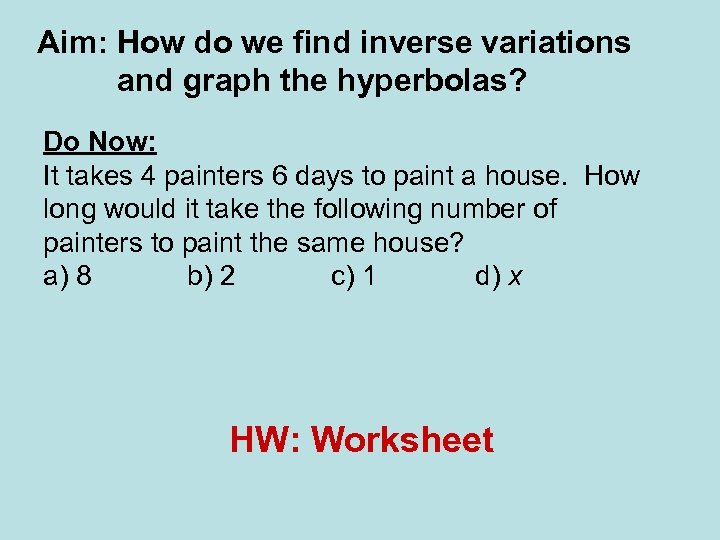Aim: How do we find inverse variations and graph the hyperbolas? Do Now: It takes 4 painters 6 days to paint a house. How long would it take the following number of painters to paint the same house? a) 8 b) 2 c) 1 d) x HW: Worksheet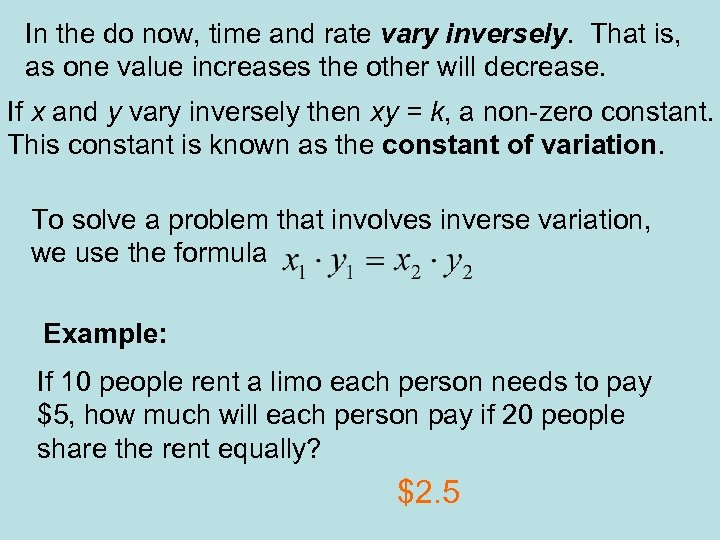In the do now, time and rate vary inversely. That is, as one value increases the other will decrease. If x and y vary inversely then xy = k, a non-zero constant. This constant is known as the constant of variation. To solve a problem that involves inverse variation, we use the formula Example: If 10 people rent a limo each person needs to pay \$5, how much will each person pay if 20 people share the rent equally? \$2. 5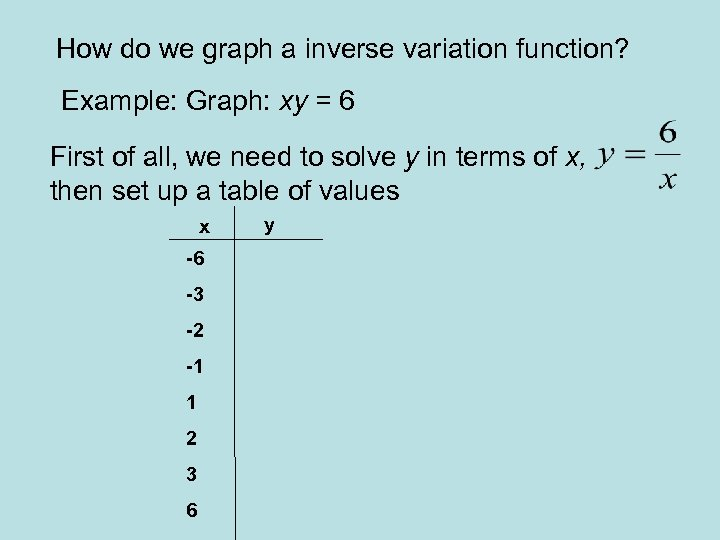How do we graph a inverse variation function? Example: Graph: xy = 6 First of all, we need to solve y in terms of x, then set up a table of values x -6 -3 -2 -1 1 2 3 6 y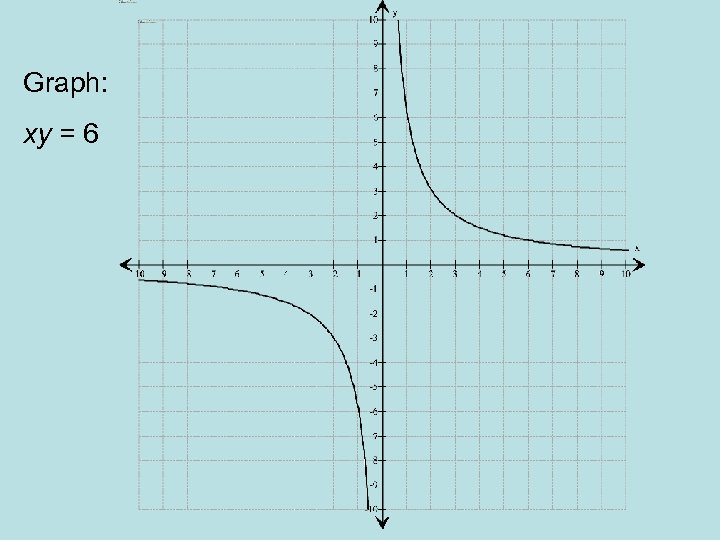Graph: xy = 6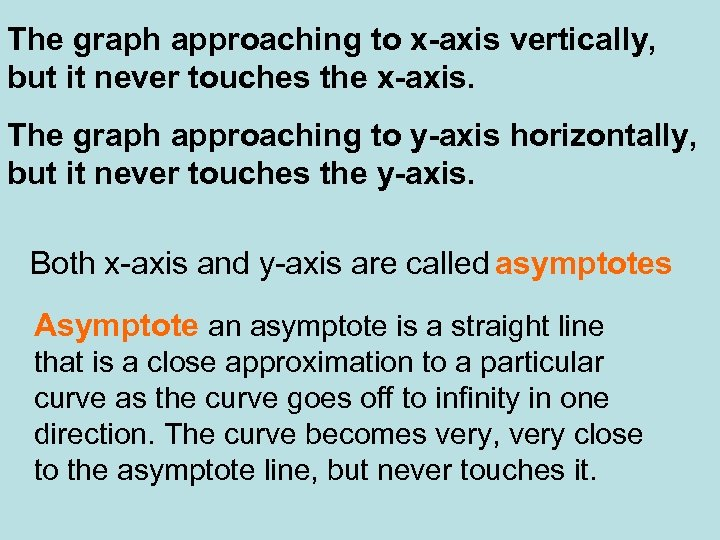The graph approaching to x-axis vertically, but it never touches the x-axis. The graph approaching to y-axis horizontally, but it never touches the y-axis. Both x-axis and y-axis are called asymptotes Asymptote an asymptote is a straight line that is a close approximation to a particular curve as the curve goes off to infinity in one direction. The curve becomes very, very close to the asymptote line, but never touches it.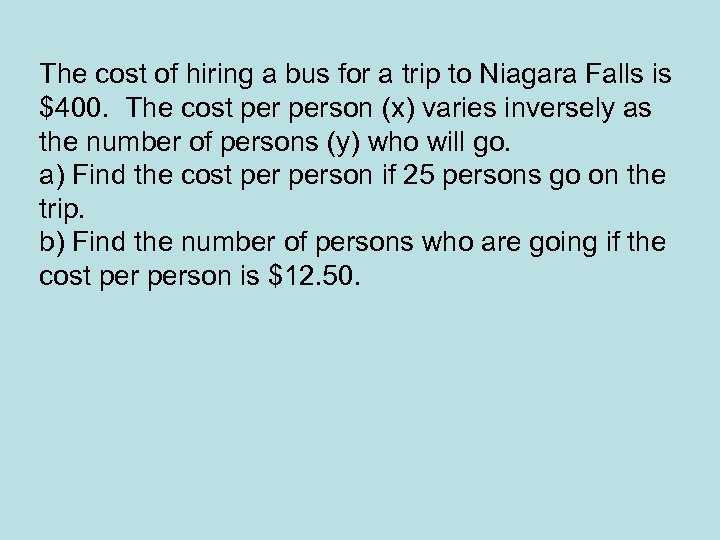The cost of hiring a bus for a trip to Niagara Falls is \$400. The cost person (x) varies inversely as the number of persons (y) who will go. a) Find the cost person if 25 persons go on the trip. b) Find the number of persons who are going if the cost person is \$12. 50.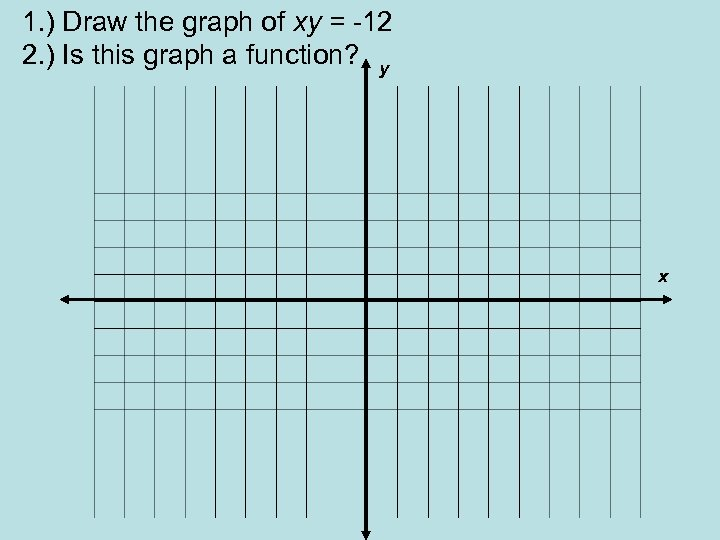1. ) Draw the graph of xy = -12 2. ) Is this graph a function? y x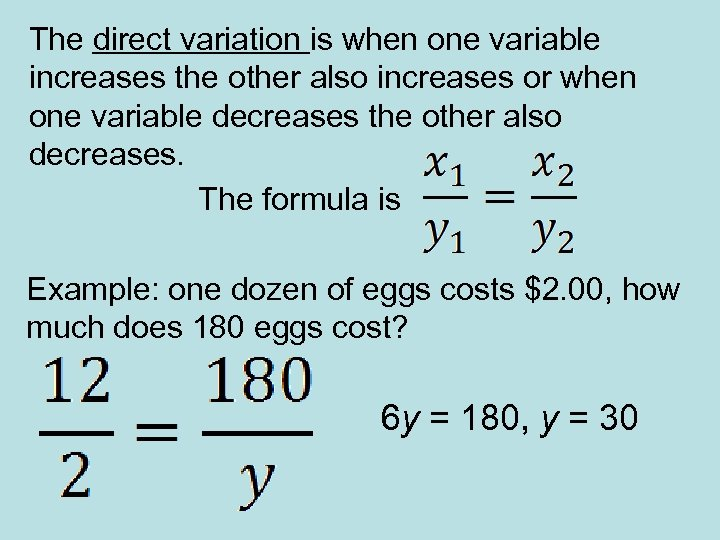The direct variation is when one variable increases the other also increases or when one variable decreases the other also decreases. The formula is Example: one dozen of eggs costs \$2. 00, how much does 180 eggs cost? 6 y = 180, y = 30